Next: 3.1.3 The Hydrodynamic Transport Up: 3.1 Sets of Partial Previous: 3.1.1 The Basic Semiconductor

## 3.1.2 The Drift-Diffusion Transport Model

The drift-diffusion current relations can, amongst others, be derived from the Boltzmann transport equation by the method of moments or from basic principles of irreversible thermodynamics under the assumption T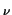= TL. The electron and hole current densities are given by

 Jn = q .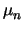. n .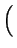grad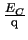-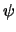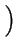+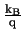.. grad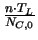, (3.5)

 Jp = q .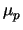. p .grad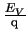--.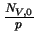. grad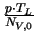. (3.6)

Here,anddenote the carrier mobilities, and TL is the lattice temperature. These current relations account for position-dependent band edge energies, EC and EV, and position-dependent effective masses, which are included in the effective density of states, NC, 0 and NV, 0. The index 0 indicates that NC, 0 and NV, 0 are evaluated at some (arbitrary) reference temperature, T0, which is constant in real space regardless of what the local values of the lattice and carrier temperatures are.Next: 3.1.3 The Hydrodynamic Transport Up: 3.1 Sets of Partial Previous: 3.1.1 The Basic Semiconductor
Tibor Grasser
1999-05-31